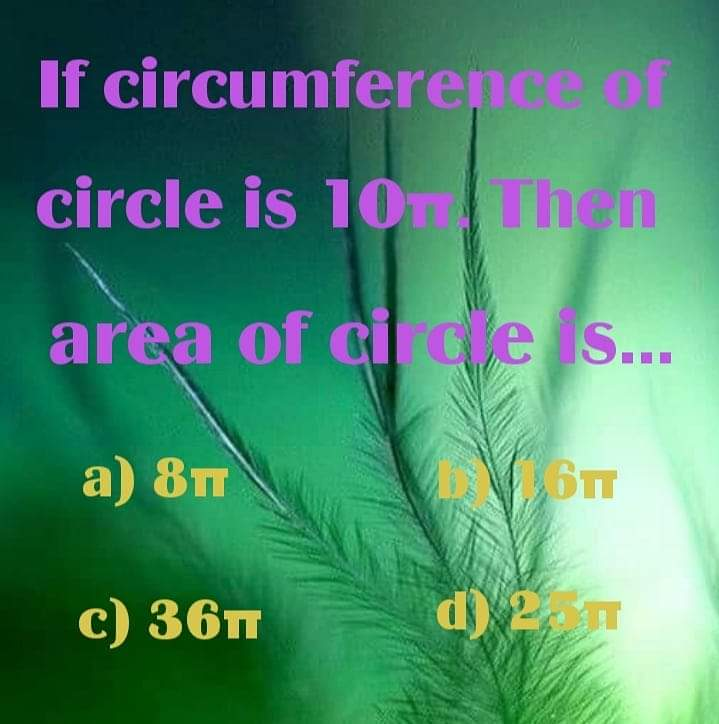# Find Area of Circle

• MHB
mathlandMy Effort:

Circumference = pi•d

10 •pi = pi•d

10•pi/pi = d

10 = d, where d is the diameter of the circle.

Area = pi•r^2, where r is the radius of the circle.

Diameter = 2 times the radius.

10pi = 2r

10pi/2 = r

5pi = r

A = pi•r^2

A = pi(5pi)^2

A = 25•pi^3, which makes no sense.

Only the volume is cubed. This is not a volume question.

Gold Member
MHB
Circumference = pi•d
10 •pi = pi•d
10•pi/pi = d
10 = d, where d is the diameter of the circle. Correct!
Area = pi•r^2, where r is the radius of the circle.
Diameter = 2 times the radius.
10pi = 2r Wrong!
The diameter is 10, not 10pi.

mathland
The diameter is 10, not 10pi.

I see my error. Thanks.